### Home > CALC > Chapter Ch5 > Lesson 5.2.1 > Problem5-57

5-57.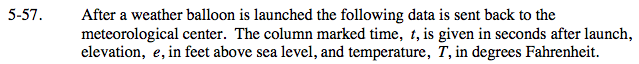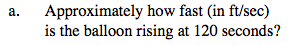Since we cannot find the derivative (IROC), calculate the average rate of change.
For e(t):

$\text{You could use Hana's method: }\frac{e(150)-e(120)}{150-120}$

$\text{or Anah's method: }\frac{e(120)-e(90)}{120-90}$

$\text{or Hanah's method: }\frac{e(150)-e(90)}{150-90}$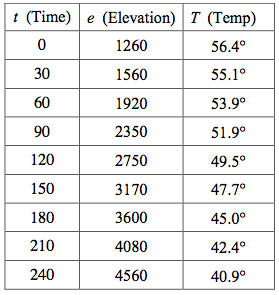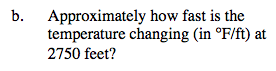Use Hana, Anah, or Hanah's method to approximate the rate of change for T(e) at e = 2750.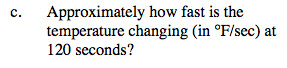Use Hana, Anah, or Hanah's method to approximate the rate of change for T(t) at t = 120.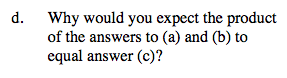$\text{Rewrite: }e'(t)=\frac{de}{dt} \text{ and }T'(e)=\frac{dT}{de}$

$\frac{de}{dt}\cdot \frac{dT}{de}=$